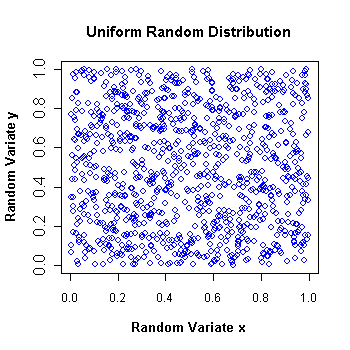I have forgotten

•http://facebook.com/
•https://www.google.com/accounts/o8/id
•https://me.yahoo.comCOST (GBP)1.980.000

# LGM

Random number generator class using the Lewis-Goodman-Miller algorithm with additional Marsaglia mixing.
Controller: CodeCogsContentsC++

## Class LGM

This class produces good quality random numbers using the Lewis-Goodman-Miller algorithm. It is best suited for simulations which require random sequences of length less than 10 million.

To give you an idea of the running time for each of the functions, here are the results for generating 100,000,000 random numbers on a 750MHz microprocessor :
• genReal() 11 seconds
• genInt() 11 secondsThis diagram is obtained by generating two sequences of 1000 uniform floating point random numbers in the (0, 1) interval and then plotting them one versus the other. It shows that the distribution is indeed uniform.

## References:

• Numerical Recipes in C by Press, Flannery, Teukolsky, Vetterling published by the Cambridge University Press.
• The Newran03 random number generator library of Robert Davies, http://www.robertnz.net/nr03doc.htm

### Example 1

The following example displays 20 random floating point numbers and 20 random large integers. It uses two different generators to achieve this. The first generator uses the system timer to initialize the seed, while the second is simply initialized with a particular value. Notice that it was necessary to divide the timer with the LGMDIV value in order to keep the seed in the (0, 1) interval. The output of the first generator will obviously vary with each execution of the program, while the output of the second will always be the same if the seed is never changed.
```#include <iostream>
#include <time.h>

#include <codecogs/statistics/random/lgm.h>
using namespace std;

#define LGMDIV 2147483648.0

int main() {
Stats::Random::LGM A(time(0) / LGMDIV);
Stats::Random::LGM B(0.113);

for (int i = 0; i < 20; ++i)
cout << A.genReal() << endl;
cout << endl;

for (int i = 0; i < 20; ++i)
cout << B.genInt() << endl;
return 0;
}```

### Authors

Lucian Bentea (August 2005)
##### Source Code

Source code is available when you agree to a GP Licence or buy a Commercial Licence.

Not a member, then Register with CodeCogs. Already a Member, then Login.

## Members of LGM

#### LGM

 LGM( double s` = 0.46875` )[constructor]
 s Default value = 0.46875

#### SetSeed

 voidsetSeed( double s )
 s Seed must be in the (0, 1) interval (endpoints are excluded).